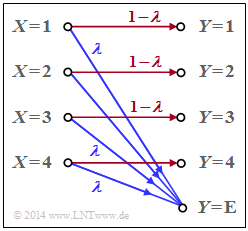# Exercise 3.11: Erasure ChannelErasure channel with
four inputs and five outputs

An erasure channel is considered with

• the  $M$  inputs  $x ∈ X = \{1,\ 2, \ \text{...} \ ,\ M\}$,  and
• the  $M + 1$  outputs  $y ∈ Y = \{1,\ 2,\ \ \text{...} \ ,\ M,\ \text{E}\}.$

The graph shows the model for the special case  $M = 4$.  The sink symbol  $y = \text{E}$  takes into account an erasure for the case that the receiver cannot make a sufficiently certain decision.

The transition probabilities are given for  $1 ≤ μ ≤ M$  as follows:

$${\rm Pr}(Y \hspace{-0.05cm} = \mu\hspace{-0.05cm}\mid \hspace{-0.05cm} X \hspace{-0.05cm}= \mu) = 1-\lambda \hspace{0.05cm},$$
$${\rm Pr}(Y \hspace{-0.05cm} = {\rm E}\hspace{-0.05cm}\mid \hspace{-0.05cm} X \hspace{-0.05cm}= \mu) = \lambda \hspace{0.05cm}.$$

We are looking for:

• the capacity  $C_{M\rm –EC}$  of this M–ary Erasure Channel,
• the capacity  $C_{\rm BEC}$  of the  Binary Erasure Channels  as a special case of the above model.

Hints:

### Questions

1

What  $P_X(X)$  should be generally applied for the channel capacity calculation?

 $P_X(X) = (0.5, \ 0.5),$ $P_X(X) = (1/M,\ 1/M, \ \text{...} \ ,\ 1/M),$ $P_X(X) = (0.1,\ 0.2,\ 0.3,\ 0.4).$

2

How many probabilities  $p_{μκ} = {\rm Pr}\big[(X = μ) ∩ (Y = κ)\big]$  are nonzero?

 Exactly  $M · (M + 1)$, Exactly  $M$, Exactly  $2 · M$.

3

What is the sink entropy in general and for  $M = 4$  and  $λ = 0.2$?

 $H(Y) \ = \$ $\ \rm bit$

4

Calculate the irrelevance.  What is the value for  $M = 4$  and  $λ = 0.2$?

 $H(Y|X) \ = \$ $\ \rm bit$

5

What is the channel capacity  $C$  as a function of  $M$?

 $M = 4\text{:} \hspace{0.5cm} C\ = \$ $\ \rm bit$ $M = 2\text{:} \hspace{0.5cm} C\ = \$ $\ \rm bit$

6

What is the channel capacity of the BEC channel in compact form?

 $C_{\rm BEC} = 1 - λ,$ $C_{\rm BEC} = 1 - H_{\rm bin}(λ).$

### Solution

#### Solution

(1)  Correct is the proposed solution 2:

• Due to the symmetry of the transition probabilities  $P_{Y|X}(Y|X)$  it is obvious that a uniform distribution will lead to the maximum mutual information  $I(X; Y)$  and therefore to the channel capacity  $C$ :
$$P_X(X) = P_X\big ( \hspace{0.03cm}X\hspace{-0.03cm}=1\hspace{0.03cm}, \hspace{0.08cm} X\hspace{-0.03cm}=2\hspace{0.03cm},\hspace{0.08cm}\text{...}\hspace{0.08cm}, X\hspace{-0.03cm}=M\hspace{0.03cm}\big ) = \big [\hspace{0.03cm}1/M\hspace{0.03cm}, \hspace{0.08cm} 1/M\hspace{0.03cm},\hspace{0.03cm}\text{...}\hspace{0.08cm},\hspace{0.08cm} 1/M\hspace{0.03cm}\big ]\hspace{0.05cm}.$$
• In the special case  $M = 2$  also  $P_X(X) = (0.5, \ 0.5)$  would be correct.

(2)  Correct is the proposed solution 3, i.e. exactly  $2M$  connections.  Because:

• From each source symbol  $X = μ$  one gets to the sink symbol  $Y = μ$  as well as to the erasure  $Y = \text{E}$.

(3)  All probabilities  ${\rm Pr}(Y = 1), \hspace{0.05cm} \text{...}\hspace{0.05cm} , \hspace{0.08cm}{\rm Pr}(Y = M)$  are equal.  Thus, for  $μ = 1, \hspace{0.05cm} \text{...} \hspace{0.05cm} , \hspace{0.08cm} M$ we obtain:

$${\rm Pr}(Y \hspace{-0.05cm} = \mu) = ( 1-\lambda)/M \hspace{0.05cm}.$$
• Moreover, from each source symbol  $X = 1, \hspace{0.05cm} \text{...}\hspace{0.05cm} , X = M$  one also gets to the erasure  $Y = \text{E}$:
$${\rm Pr}(Y \hspace{-0.05cm} = {\rm E}) = \lambda \hspace{0.05cm}.$$
• Inspection reveals that the sum of all  $M + 1$  sink symbol probabilities actually adds up t  $1$ .
• It follows for the sink entropy:
$$H(Y) = M \cdot \frac{ 1-\lambda }{M} \cdot {\rm log}_2 \hspace{0.1cm} \frac{M}{1 - \lambda} \hspace{0.15cm}+\hspace{0.15cm} \lambda \cdot {\rm log}_2 \hspace{0.1cm} \frac{1}{\lambda} \hspace{0.05cm}.$$
• Summarized with the binary entropy function, this gives:
$$H(Y) = (1-\lambda) \cdot {\rm log}_2 \hspace{0.1cm} M \hspace{0.15cm}+\hspace{0.15cm} H_{\rm bin} (\lambda ) \hspace{0.05cm}$$
and with  $M = 4$   as well as  $λ = 0.2$:
$$H(Y) = 1.6 \,{\rm bit} + H_{\rm bin} (0.2 ) \hspace{0.15cm} \underline {=2.322\,{\rm bit}} \hspace{0.05cm}.$$

(4)  The  $2M$  joint probabilities

$${\rm Pr} \big[(X = μ) ∩ (Y = κ)\big] ≠ 0$$
and the conditional probabilities
$$pκ|μ = {\rm Pr}(Y = κ|X = μ)$$
show the following properties:
1.   The combination  $p_{μκ} = (1 – λ)/M$  and  $p_{κ|μ} = 1 – λ$  occurs  $M$  times.
2.   The combination  $p_{μκ} = λ/M$  and  $p_{κ|μ} = λ$  also occurs $M$ times.

It follows that:

$$H(Y \hspace{-0.15cm}\mid \hspace{-0.15cm} X) \hspace{-0.01cm} =\hspace{-0.01cm} M \cdot \frac{ 1-\lambda }{M} \cdot {\rm log}_2 \hspace{0.1cm} \frac{1}{1 - \lambda} \hspace{0.15cm}+\hspace{0.15cm}M \cdot \frac{ \lambda }{M} \cdot {\rm log}_2 \hspace{0.1cm} \frac{1}{ \lambda} = ( 1-\lambda) \cdot {\rm log}_2 \hspace{0.1cm} \frac{1}{1 - \lambda} \hspace{0.15cm}+\hspace{0.15cm} \lambda \cdot {\rm log}_2 \hspace{0.1cm} \frac{1}{ \lambda} = H_{\rm bin} (\lambda)\hspace{0.05cm}.$$
• The result is independent of  $M$.  With  $λ = 0.2$  we obtain:
$$H(Y \hspace{-0.1cm}\mid \hspace{-0.1cm} X) = H_{\rm bin} (0.2 ) \hspace{0.15cm} \underline {=0.722\,{\rm bit}} \hspace{0.05cm}.$$

(5)  The channel capacity  $C$  is equal to the maximum mutual information  $I(X; Y)$,  where the maximization with respect to  $P_X(X)$  has already been considered by the symmetric approach:

$$C = \max_{P_X(X)} \hspace{0.15cm} I(X;Y) = H(Y) - H(Y \hspace{-0.1cm}\mid \hspace{-0.1cm} X) = ( 1-\lambda) \cdot {\rm log}_2 \hspace{0.1cm} M + H_{\rm bin} (\lambda) - H_{\rm bin} (\lambda) = ( 1-\lambda) \cdot {\rm log}_2 \hspace{0.1cm} M \hspace{0.05cm}$$
$$\Rightarrow \hspace{0.3cm} M = 4\text{:} \hspace{0.3cm} \underline {C=1.6\,\,{\rm bit}} \hspace{0.05cm}, \hspace{0.8cm} M = 2\text{:} \hspace{0.3cm} \underline {C=0.8\,\,{\rm bit}} \hspace{0.05cm}.$$

(6)  The  "Binary Erasure Channel"  $\rm (BEC)$  is a special case of the general model considered here with  $M = 2$:

$$C_{\rm BEC} = 1-\lambda \hspace{0.05cm}.$$
• Thus, the correct solution proposal 1.
• The second proposed solution, on the other hand, applies to the  "Binary Symmetric Channel"  $\rm (BSC)$  with distortion probability  $λ$.